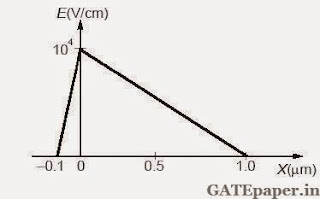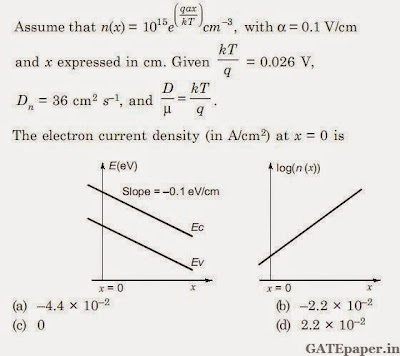### GATE 2015 ECE Electron Devices (EDC) - Video Solutions

GATE 2015 (Electron Devices)

1. Which one of the following processes is preferred to form the gate dielectric (SiO2) of MOSFET?
a. Sputtering
b. Molecular Beam Epitaxy
c. Wet Oxidation
d. Dry Oxidation

2. In the circuit shown, the BJT has a current gain (β) of 50. For an emitter base voltage VEB = 600 mV, the emitter collector voltage VEC (in volts) is_______________3. If the base width in a bipolar junction transistor is doubled, which one of the following statements will be TRUE?
a. Current gain will increase
b. Unity gain frequency will increase
c. Emitter base junction capacitance will increase
d. Early voltage will increase

4. In the circuit shown, assume that the diodes D1 and D2 are ideal. The average value of voltage Vab (in volts), across terminals a and b is ________________5. The electric field profile in the depletion region of a PN junction in equilibrium is shown in the figure. Which one of the following is NOT TRUE?a. The left side of the junction is N-type and the right side is P-type
b. Both the N-type and P-type depletion regions are uniformly doped
c. The potential difference across the depletion region is 700 mV
d. If the P-type region has a doping concentration of 1015 cm-3, then the doping concentration in the N-type region will be 1016 cm-3

6. In the circuit shown, the both the enhancement mode NMOS transistors have the following characteristics: Kn = µn.Cox(W/L) = 1 mA/V2, VTN = 1 volt.Assume that the channel length modulation parameter λ is zero and body is shorted to source. The minimum supply voltage VDD (in volts) needed to ensure that transistor M1 operates in saturation mode of operation is ___________________

7. The current in an enhancement mode NMOS transistor biased in saturation mode was measured to be 1 mA at a drain to source voltage of 5 volts. When the drain – source voltage was increased to 6 volts, while keeping gate-source voltage same, the drain current increased to 1.02 mA. Assume that drain to source saturation voltages is much smaller than the applied drain source voltage. The channel length modulation parameter λ (in V-1) is __________

8. An NPN BJT having reverse saturation current Is = 10-15 A is biased in the forward active region with VBE =700 mV. The thermal voltage (VT) is 25 mV and the current gain (β) may vary from 50 to 150 due to manufacturing variations. The maximum emitter current (in µA) is ____________________________

9. A silicon sample is uniformly doped with donor type impurities with a concentration of 1016 cm-3. The electron and hole mobilities in the sample are 1200 cm2/V-sec and 400 cm2/V-sec respectively. Assume complete ionization of impurities. The charge of an electron is 1.6x10-19 C. The resistivity of the sample (in Ω-cm) is _______________

10. A region of negative differential resistance is observed in the current voltage characteristics of a silicon PN junction if
a. Both the P-region and N-region are heavily doped
b. The N-region is heavily doped compared to P-region
c. The P-region is heavily doped compared to N-region
d. An intrinsic silicon region is inserted between the P-region and the N-region

11. For the circuit shown, assume ideal diodes. The shape of the output (Vout) for the given sine wave input (Vin) will be ________________12. In the circuit shown below, the Zener diode is ideal and the Zener voltage is 6 volts. The output voltage Vo (in volts) is _______________13. For the NMOSFET in the circuit shown, the threshold voltage is Vth greater than zero. The source voltage VSS is varied from 0 to VDD. Neglecting the channel length modulation, the drain current ID as a function of VSS is represented by14. For a silicon diode with ling P and N regions, the acceptor and donor impurity concentrations are 1x1017 cm-3 and 1x1015 cm-3 respectively. The lifetimes of electron in P-region and holes in N-region are both 100 µs. The electron and hole diffusion coefficients are 49 cm2/sec and 36 cm2/sec respectively. Assume thermal voltage is 26 mV, the intrinsic carrier concentration is 1x1010 cm-3 and q = 1.6x10-19 C. When a forward voltage of 208 mV is applied across the diode, the hole current density (in nA/cm2) injected from P-region to N-region is ___________

15. A MOSFET in saturation has a drain current of 1 mA for VDS = 0.5 volts. If the channel length modulation coefficient is 0.05 V-1, the output resistance (in kΩ) of the MOSFET is ________

16. The built in potential of an abrupt PN junction is 0.75 volts. If its junction capacitance (CJ) at a reverse bias (VR) of 1.25 volts is 5 pF. The value of CJ (in pF) when VR = 7.25 volts is _____

17. A piece of silicon is doped uniformly with phosphorous with a doping concentration of 1016 per cm3. The expected value of mobility verses doping concentration for silicon assuming full dopant ionization is shown below. The charge of an electron is 1.6x10-19 C. The conductivity (in Simons/cm) of the silicon sample at 300oK is _______________18. An N-type silicon sample is uniformly illuminated with light which generates 1020 electron-hole pairs per cm3 per second. The minority carrier lifetime in the sample is 1 µs. In the steady state, the hole concentration in the sample is approximately 10x, where x is an integer. The value of x is _________________

19. In MOS capacitor with an oxide layer thickness of 10 nm. The maximum depletion layer thickness is 100 nm. The permittivity’s of the semiconductor and the oxide layer are εs and εox respectively. Assuming εsox = 3, the ratio of the maximum capacitance to the minimum capacitance of this MOS capacitor is ____________

20. The energy band diagram and the electron density profile n(x) in a semiconductor are shown in the figures.21. A DC voltage of 10 volts is applied across an N-type silicon bar having a rectangular cross section and length of 1 cm as shown. The donor doping concentration ND and the mobility of electron µn are 1016 cm-3 and 1000 cm2/V-sec respectively. The average time (in µs) taken by the electrons to move from one end of the bar to the other end is _______________22. The diode in the circuit given below has VON = 0.7 volts but is ideal otherwise. The current (in mA) in the 4 kΩ resistor is __________________